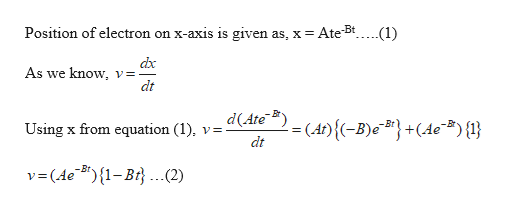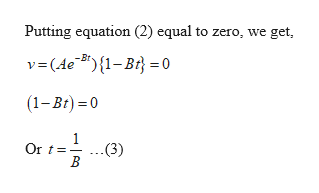An electron moving along the x-axis has a position given by x = Ate - Bt m, where t is in seconds and A and B are positive constants. In terms of A and B, how far is the electron from the origin when it momentarily stops?

Question

An electron moving along the x-axis has a position given by x = Ate - Bt m, where t is in seconds and A and B are positive constants. In terms of A and B, how far is the electron from the origin when it momentarily stops?

Step 1

Given:

Position of electron on x-axis, x = Ate-Bt

A and B are the postive constants.

Step 2

Calculating the velocity of the electron:help_outlineImage TranscriptionclosePosition of electron on x-axis is given as, x Ate5t.... .(1) dx As we know, v=- dt d(Ate (At){(-B)#} +(4c"#){} Using x from equation (1) y= dt v=(AeB") {1-Bi. ..2) V fullscreen
Step 3

Calculating the time at which the electron momentarily stops:

To s...help_outlineImage TranscriptionclosePutting equation (2) equal to zero, we get, v(Ae B){1-Br0 (1-Br) 0 Or t (3) fullscreen

Want to see the full answer?

See Solution

Want to see this answer and more?

Our solutions are written by experts, many with advanced degrees, and available 24/7

See Solution
Tagged in

Physics